# VLOOKUP w/o #N/A Error – IFERROR / IFNA – Excel & Google Sheets

This tutorial will teach you how to handle VLOOKUP errors with IFERROR and IFNA in Excel and Google Sheets.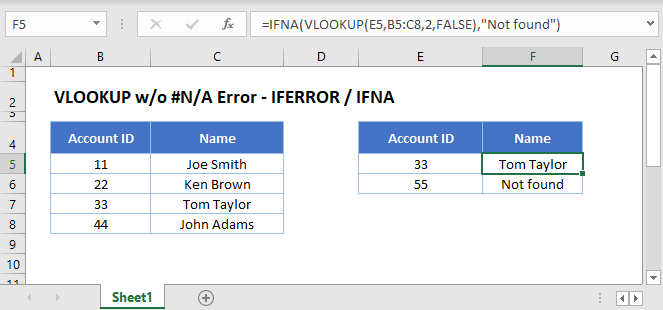## IFERROR and VLOOKUP

You can use VLOOKUP with the IFERROR Function to return a value (or other calculation) instead of an error if an error occurs..

This example will perform a second VLOOKUP if the first results in an error.

``=IFERROR(VLOOKUP(F3,B3:D6,3,FALSE),VLOOKUP("Else",B3:D6,3,FALSE))``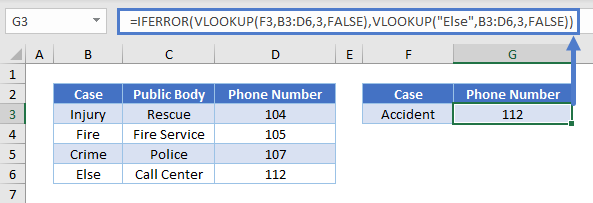In the example above, we look for a Case that does not exist in the lookup table. Without the IFERROR Function the VLOOKUP Function would return a #N/A error. Using the IFERROR Function we can replace the error by another lookup for a general case. In other words, if the Case is not “Injury,” “Accident,” or “Crime,” look up the Phone Number for the catchall case category “Else.”

Please note that the IFERROR Function catches all kinds of errors: #N/A, #VALUE!, #REF!, #DIV/0!, #NUM!, #NAME?. This does not always produce the result you want. In the below example our lookup table is a named range: LookupTable. If we accidentally mistype its name (e.g. “Lookup Table”), the formula will return a “Not found” message, even for those account IDs that are in the list.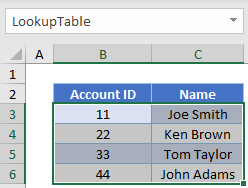``=IFERROR(VLOOKUP(E3,Lookup Table,2,FALSE),"Not found")``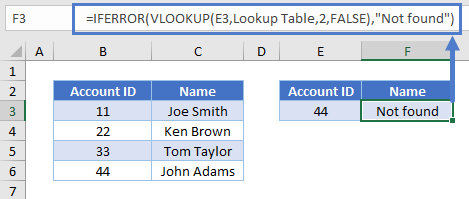Since we mistyped the name of the lookup table, the VLOOKUP Function returns a #NAME? error. With the IFERROR Function we replace all types of errors with the “Not found” message, which is misleading.

If you want to handle only #N/A errors, use the IFNA Function instead.

## IFNA and VLOOKUP

When you search for a value with the VLOOKUP Function that does not exist in your lookup array, you will get an #N/A error. With the IFNA Function you can change the error display to an arbitrary message (or even an empty string).

``=IFNA(VLOOKUP(E3,B3:C6,2,FALSE),"Not found")``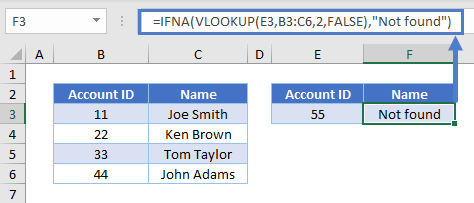There is no Account ID 55, so the formula gives us “Not found.”

Looking back to our example where we mistyped the name of the data range, using IFNA instead of IFERROR tells us we’ve made a mistake and not that the value wasn’t found: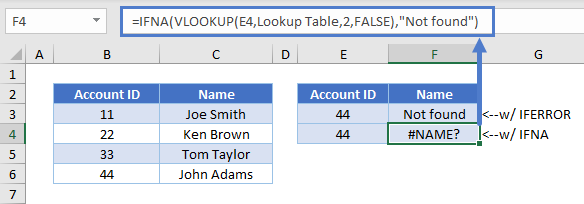The #NAME? error means there’s something wrong with the syntax of our formula, so we need to review and correct what we typed in.

## VLOOKUP w/o #N/A Error in Google Sheets

These formulas work in exactly the same way in Google Sheets as in Excel.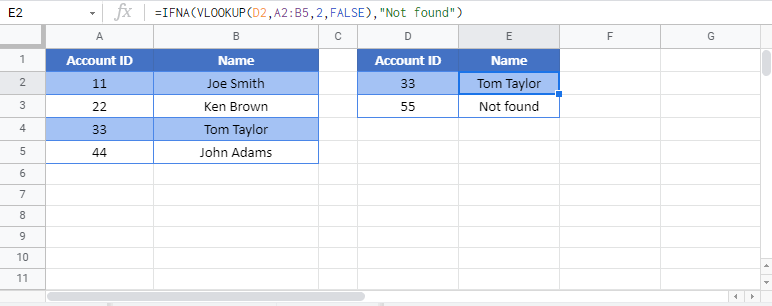### Excel Practice Worksheet

Practice Excel functions and formulas with our 100% free practice worksheets!